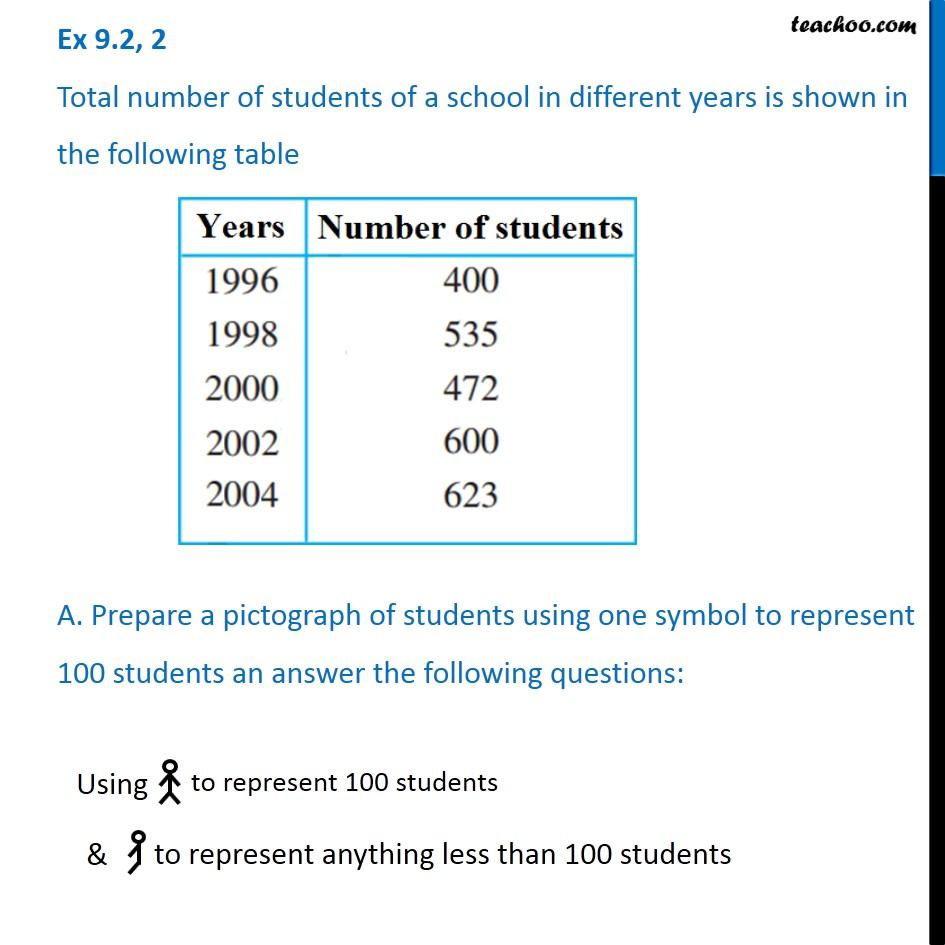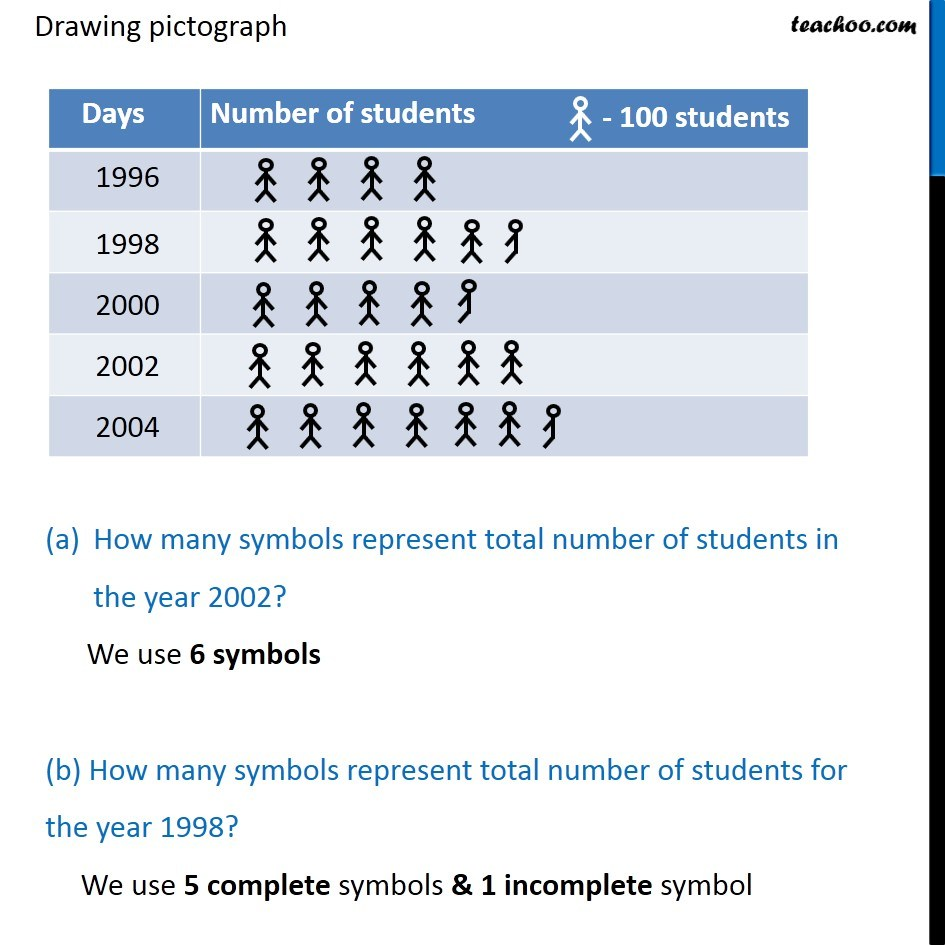Drawing a pictograph

Chapter 9 Class 6 Data Handling
Concept wiseSolve all your doubts with Teachoo Black (new monthly pack available now!)

### Transcript

Ex 9.2, 2 Total number of students of a school in different years is shown in the following table A. Prepare a pictograph of students using one symbol to represent 100 students an answer the following questions: Using to represent 100 students to represent anything less than 100 students Drawing pictograph How many symbols represent total number of students in the year 2002? We use 6 symbols (b) How many symbols represent total number of students for the year 1998? We use 5 complete symbols & 1 incomplete symbol Drawing pictograph How many symbols represent total number of students in the year 2002? We use 6 symbols (b) How many symbols represent total number of students for the year 1998? We use 5 complete symbols & 1 incomplete symbol